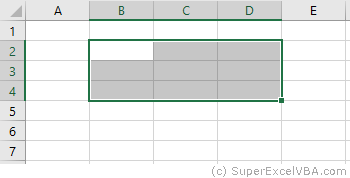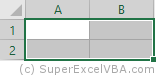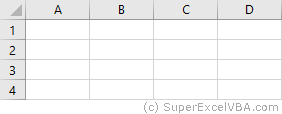# Resizing VBA Range

To create a Range of cells from parameters or another Range of cells, it is required knowledge of resizing and/or displacement commands. The main ones being Resize and Offset.

## VBA Offset

Offset is a command for moving, either horizontally or vertically, a Range of cells.

    Range("B2").Offset(RowOffSet:=1, ColumnOffset:=1).Select
'The above command is the same as Range ("B2"). Offset (1, 1) .Select


The above code will select cell "C3".

• RowOffSet: integer that shifts rows downwards as it value increases
• ColumnOffset: integer the shifts columns to the right as its value increases

It is not necessary to use the terms: RowOffSet and ColumnOffSet. Just as in a function, you can use only the numbers as parameters (in the order RowOffSet and then ColumnOffset), making the parameterization implicit.

  Range("B2").Offset(-1, -1).Select


The above code will select cell "A1", since negative numbers mean retreating rows or columns.

The advantage of explicit parameterization (using the RowOffSet and ColumnOffSet terms) is that it allows you to change the order in which the parameters are written.

  Range("B2").Offset(2, 3).Select
Range("B2").Offset(ColumnOffset:=3, RowOffSet:=2).Select
'Both of the above commands perform the same action


A Range can contain more than one cell, and this range can be shifted with Offset.

    Range("B2:D4").Offset(1, 1).Select


The above code will select the cells from "C3" to "E5", starting from the reference range from "B2" to "D4".## VBA Resize

Resize is a command for creating a new Range starting from the lowest-row cell and the lowest-column of the reference Range (even if it has only one cell).

    Range("A1").Resize(RowSize:=2, ColumnSize:=2).Select
'The above command is the same as Range ("A1"). Resize (2, 2) .Select


The above code will select the cells from "A1" to "B2".

• RowSize: integer that determines the amount of rows to expand from the lowest row and column cell to form a Range (expanding from top to bottom).
• ColumnSize: integer that determines the amount of columns to expand from the lowest row and column cell to form a Range (expanding from left to right).

In the same way as the Offset command, you can omit the parameters name (RowSize, ColumnSize).

  Range("B2").Resize(3, 3).Select


The above code will select the cells from "B2" to "D4".

The Resize property will always use the lower-line and lower-column cell as the starting point. The size of the Range reference is irrelevant:

  Range("A1:J20").Resize(2, 2).Select


In this selection the lower-line and lower-column cell will be "A1". Thus, the code will then select the cells from "A1" to "B2".Unlike the Offset command, Resize does not accept negative values.

## VBA Resize along with VBA Offset

You can use the Resize and the Offset commands together in a single line of code:

    Range("A2:B3").Select
Range("A2:B3").Resize(1, 2).Offset(-1, 1).Select
Range("A2:B3").Offset(-1, 1).Resize(1, 2).Select 'Alternative to the previous lineAlthough in this case the final Range is the same, the order of execution resolves from left to right.

    Range("A2:B3").Resize(1, 2).Offset(-1, 1).Select
'Execution order: First Range("A2:B3").Resize(1, 2) and then .Offset(-1, 1) for later .Select